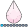## Proc Phreg for time varying exposure variable- different output

Hi,

My data set looks like:

 ID event Met_01 Start (days) Stop (days) Fu_time (stop-start) 1 0 0 0 230 230 1 1 1 230 1430 1200 2 1 0 0 907 907 3 0 0 0 365 365 3 1 1 365 790 425

When I am applying time varying Cox regression in SAS using following 2 codes then I am getting completely different Hazard Ratio (model 1: crude HR 0.95 and model 2:crude HR 0.74).

Model 1:

proc phreg data=test;

class met_01/ref=first;

model (start, stop) * event (0) = met_01/ties=EFRON RL;

run;

Model 2:

proc phreg data=test;

class met_01/ref=first;

model fu_time* event (0) = met_01/ties=EFRON RL;

run;

I am not sure which one is to consider. Any help on this will be highly appreciated. I am struggling on this point from a long time.

1 ACCEPTED SOLUTION

Accepted SolutionsJacobSimonsen
Barite | Level 11

## Re: Proc Phreg for time varying exposure variable- different output

Both can be correct, but most likely you should use (start, stop).

It has to do with how you define the baseline hazard. If the baseline hazard should be a function of time since 0 then use (start, stop).

If the hazard functions should be reset, such it is a functio of time since last stop time then you can use your model 2.

In far most cases, model 1 is the right way to go.

For your second question about how the hazard ratio is calculated, the simple answer is that it is the maximum point of the Cox's partial likelihood function. The hazard ratio has no closed analytical form. Except in simple cases with very few events. In your case the estimated hazard ratio is the maximum point of the function (x/(1+2*x))*(1/(1+x)) which is sqrt(1/2). I dont get exactly same number as you mention btw, but I have tried run the SAS code and the solution is indeed sqrt(0.5)=0.707.

4 REPLIES 4OsoGris
SAS Employee

## Re: Proc Phreg for time varying exposure variable- different output

I think you should go with the start, stop counting-process syntax.   Your data are already in that form.

thanks!

## Re: Proc Phreg for time varying exposure variable- different output

Does anyone know what is the formulae that SAS used at the back end to output Hazard Ratio from these 2 models? Thanks.JacobSimonsen
Barite | Level 11

## Re: Proc Phreg for time varying exposure variable- different output

Both can be correct, but most likely you should use (start, stop).

It has to do with how you define the baseline hazard. If the baseline hazard should be a function of time since 0 then use (start, stop).

If the hazard functions should be reset, such it is a functio of time since last stop time then you can use your model 2.

In far most cases, model 1 is the right way to go.

For your second question about how the hazard ratio is calculated, the simple answer is that it is the maximum point of the Cox's partial likelihood function. The hazard ratio has no closed analytical form. Except in simple cases with very few events. In your case the estimated hazard ratio is the maximum point of the function (x/(1+2*x))*(1/(1+x)) which is sqrt(1/2). I dont get exactly same number as you mention btw, but I have tried run the SAS code and the solution is indeed sqrt(0.5)=0.707.

Discussion stats
• 4 replies
• 312 views
• 3 likes
• 3 in conversation